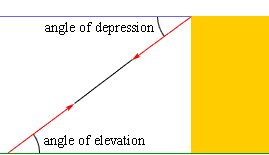Quandaries and Queries what is the difference between an angle of elevation and angle of depression? Hi Anjum, If you are looking at an object above the horizon then the angle between the horizontal and your line of sight is the angle of elevation. If you are looking at an object below the horizon then the angle between the horizontal and your line of sight is the angle of depression.In the diagram the lines of sight from the ground upwards and from the top of the building downwards are indicated in red. From the diagram you can see that the angle of elevation and the angle of depression are congruent. Penny Go to Math Central